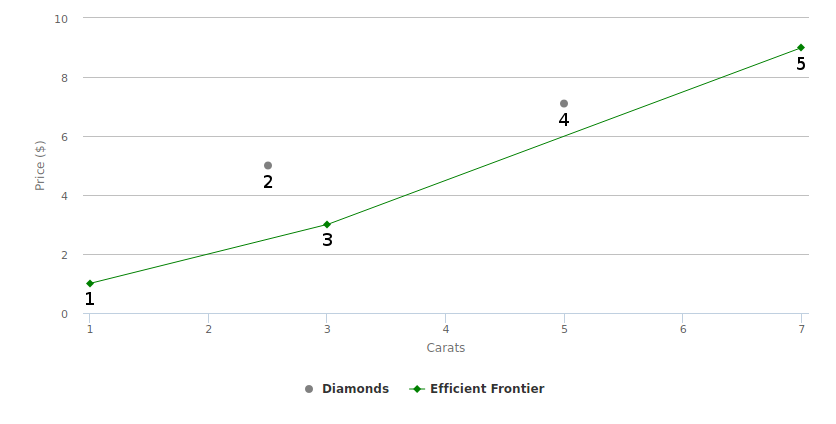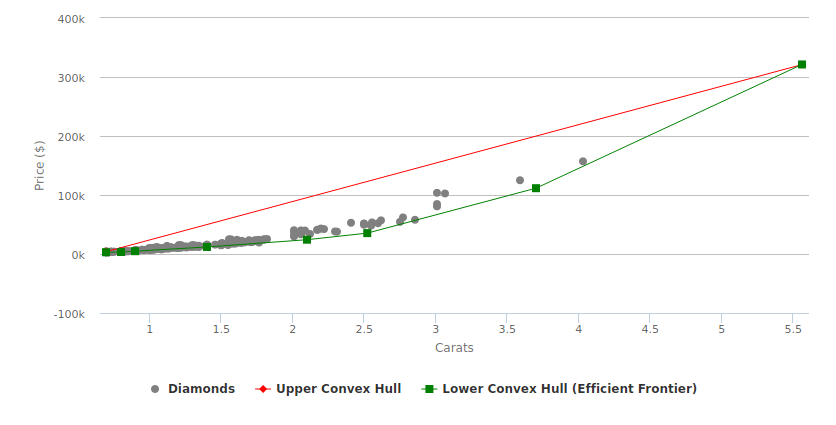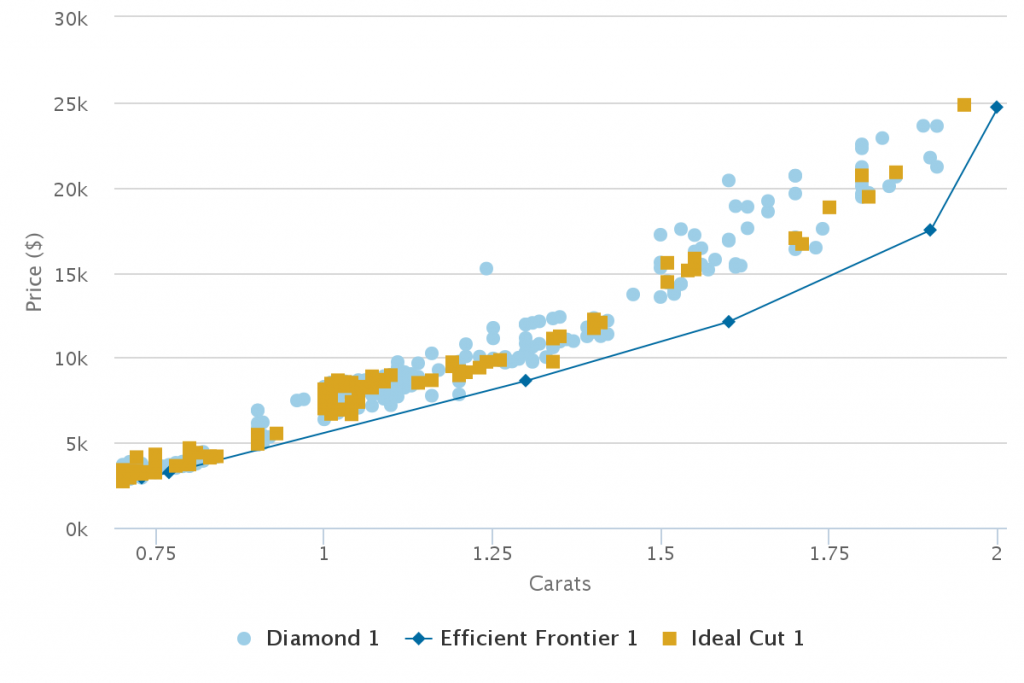# How to Find the Best Deals on Diamonds Using the Cost-Efficiency Frontier

How do you determine if a diamond is a good deal? One way is to conduct a cost-effectiveness analysis. If we graph the diamond price curves, we can apply a concept called the cost-efficiency frontier to identify the economically optimal carat/price points. The closer a diamond is to the cost-efficiency frontier, the better the deal.

## Cost-Efficiency Frontier

The cost-efficiency frontier plot compares the benefit of a diamond (carat) on the x-axis with its cost on the y-axis. In the figure below, the frontier is shown by the lines connecting diamonds 1, 3, and 5. If a diamond is above the frontier, it is not considered to be cost-effective. For example, diamond 2 is not cost-effective because it is “absolutely dominated” by diamond 3, which is both larger and cheaper than diamond 2. Similarly, diamond 4 is not cost-effective because it is “dominated in an extended fashion”; an imaginary diamond that is the weighted average of diamond 3 and diamond 5 would be larger and cheaper than diamond 4. For more information on efficiency frontier plots and dominance concepts, please see “General Methods for the Assessment of the Relation of Benefits to Costs.”The efficiency frontier is determined by calculating the convex hull of the diamond price curve. Consider the set of diamond prices below. Mathematically, the convex hull is defined as the set of all convex combinations of these points. Intuitively, imagine a rubber band wrapped tightly around all of the points; the shape it creates encloses the convex hull.

The subset of points that lie on the convex hull and support the rubber band’s shape can be partitioned into an upper (red) and lower hull (green) that are bounded by the left and right-most points in the plane. In our setup, the lower hull forms the efficiency frontier because any point above it is dominated as described above.  These points are the absolute best deals on a cost/carat basis; the closer you are to the line, the better the deal.## Choosing a Diamond

Although diamonds on the frontier are optimal on a cost/carat basis, they may not be eye-clean or have optimal proportions.  In the diamond price curves, I’ve highlighted the round diamonds with the best proportions (table size, crown angle, pavilion angle) that are cut for the shiniest light performance.  The goal is to find a diamond that catches your eye as close to the frontier as possible. I hope my tool saves you time and money in the diamond search process!